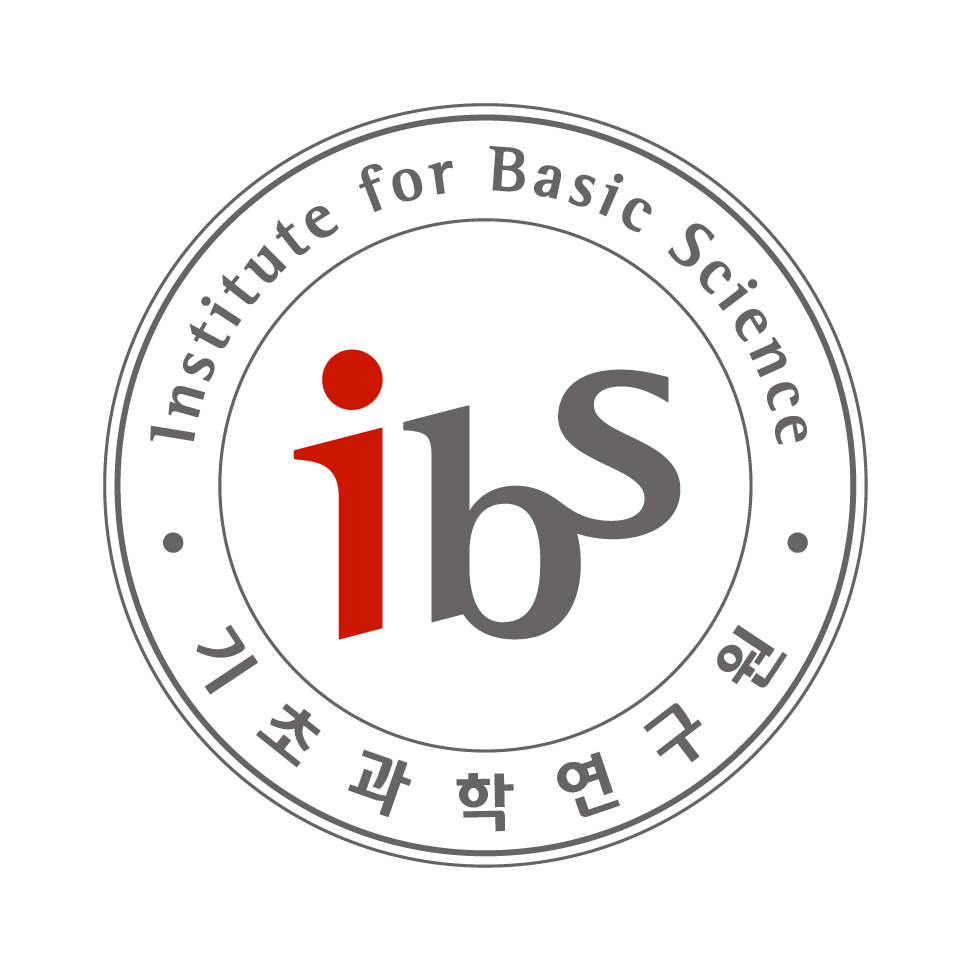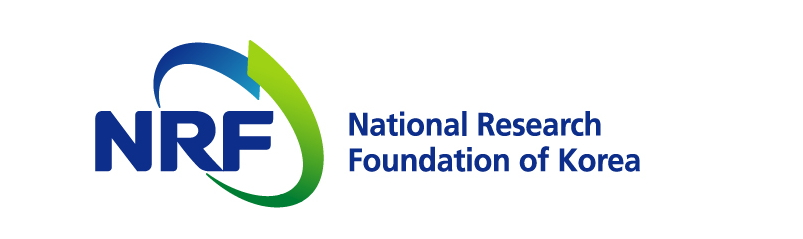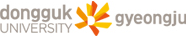# The 4th Mini Workshop on Knot theory

June 7–8, 2019

Dongguk University Gyeongju, Gyeongju, Korea

sponsered by

## Speakers

• Byung Hee An (IBS-CGP)
• Hyoungjun Kim (Ewha Womans University)
• Jung Hoon Lee (Chonbuk National University)
• Sangyop Lee (Chung-Ang University)
• Mikyoung Lim (KAIST)
• Sungjong No (Korea University)
• Seungsang Oh (Korea University)
• Alexander Stoimenow (GIST)

## Organizers

• Byung Hee An (IBS-CGP)
• Hwa Jeong Lee (DGIST)

## Talk Schedule (tentative)

June 7 (Fri) June 9 (Sat)
10:00 – 10:40 Alexander Stoimenow
10:40 – 10:50 Break
10:50 – 11:30 Sungjong No
11:30 – 13:30 Lunch
13:30 – 14:10 Arrival
and
Registration
Junghoon Lee
14:10 – 14:20 Break
14:20 – 15:00 Mikyoung Lim
15:00 – 15:30 Break
15:30 – 16:10 Seungsang Oh Sangyop Lee
16:10 – 16:20 Break
16:20 – 17:00 Hyoungjun Kim Byung Hee An
17:30 – Dinner

## Title & Abstract

Speaker
Byung Hee An (IBS-CGP)
Title
Augmentation varieties and cluster algebras for Legendrian $(2,n)$ torus knots
Abstract
For a Legendrian knot, one can associate a differential graded algebra(DGA), and any embedded exact Lagrangian filling of the knot provides an augmentation of the associated DGA. On the other hand, the space of augmentations forms an algebraic variety, called the augmentation variety. In this talk, we will provide the family of open charts for the augmentation variery of the Legendrian $(2,n)$ torus knot, which are indexed by the set of faces of the associahedron of type $A_n$ and their transition functions are given by the mutation. Moreover, each chart can be realized as the deformation space of the constructible Lagrangian filling. If time permits, we will discuss the possible generalization for $E_6$ and $E_8$ cases.
Speaker
Hyoungjun Kim (Ewha Womans University)
Title
Intrinsically chiral graphs
Abstract
The mirror symmetry of a molecule is actively researched in chemistry. The molecular structure is represented by a graph structure. Therefore, the study on the mirror symmetry of the graph is important in the natural science. A graph $G$ is said to be intrinsically chiral if every embedding of $G$ is not ambient isotopic to its mirror image. In this talk, we find two minor minimal intrinsically chiral graphs $\Gamma_7$ and $\Gamma_8$. This work is collaborated with Howon Choi and Sungjong No.

Speaker
Jung Hoon Lee (Chonbuk National University)
Title
Disk surgery on primitive disks
Abstract
For a Heegaard splitting $V \cup W$, a disk $D \subset V$ is primitive if there is a disk $E \subset W$ such that $| D \cap E | = 1$. Primitive disks are closely related to primitive elements of free groups. It is known that for a genus-$2$ Heegaard splitting of the $3$-sphere, given any two primitive disks intersecting each other, any disk surgery on one along the other yields a primitive disk. We show that for a genus-$g$ Heegaard splitting of the $3$-sphere with $g \ge 3$, there exist two intersecting primitive disks such that any disk surgery on one along the other yields no primitive disks.
Speaker
Sangyop Lee (Chung-Ang University)
Title
Torus knots with a negative single twist that yields a torus knot
Abstract
A twisted torus knot is a knot obtained from a torus knot by twisting some consecutive strands of the torus knot. More precisely, a twisted torus knot $T(p,q,r,s)$ is a torus knot $T(p,q)$ with $r$ consecutive strands $s$ times fully twisted. We determine which twisted torus knots $T(p,q,p-kq,-1)$ are a torus knot.

Speaker
Mikyoung Lim (KAIST)
Title
Series solution method for the conductivity interface problem based on the geometric function theory
Abstract
In this talk, I present the series solution method for the conductivity interface problem. For arbitrary simply connected domain in two dimensions, there exists a conformal mapping from the unit disk to the domain (as known as the Riemann mapping theorem). By reflecting the conformal mapping via a circle, we have the exterior conformal mapping as well. The series solution method is based on the geometric function theory, which is about the geometric properties of the complex analytic functions associated with a given domain, and reveals explicit relations between the interface problem solution and the inclusion’s geometry.
Speaker
Sungjong No (Korea University)
Title
Petal presentations and torus knots
Abstract
An $n$-crossing projection of knot is a projection that every crossings of the projection are $n$-tuple points. A petal projection is an $n$-crossing projection with single crossing for some $n$ that has no nesting loops. The minimum possible of $n$ is called a petal number, denoted $p(K)$. Adams introduced this concept and proved that every knot has a petal projection. In this talk, we prove that $p(K) \leq 2c(K)+1$ and find a bounds for some torus knots.

Speaker
Seungsang Oh (Korea University)
Title
Entropy of Knot Mosaics
Abstract
Knot mosaic theory was introduced by Lomonaco and Kauffman in the paper `Quantum knots and mosaics' to give a precise and workable definition of quantum knots, intended to represent an actual physical quantum system. This study is inspired by an open question about the knot mosaic enumeration suggested by them. A knot $(m,n)$-mosaic is an $(m,n)$ array of 11 mosaic tiles representing a knot or a link diagram by adjoining properly. The total number $D(m,n)$ of knot $(m,n)$-mosaics, which indicates the dimension of the Hilbert space of the quantum knot system, is known to grow in a quadratic exponential rate. Recently, the first author showed the existence of the knot mosaic constant $\delta = \lim_{m, n \rightarrow \infty} D(m,n)^{\frac{1}{mn}}$ and proved $4 \leq \delta \leq \frac{5+ \sqrt{13}}{2} = 4.302\cdots$ by developing an algorithm producing the exact enumeration of knot mosaics, which uses a recursion formula of state matrices. In this paper, we give a simpler proof of the lower bound and improve the upper bound of the knot mosaic constant as $4 \leq \delta \leq 4.113\cdots$ by introducing two new concepts: quasimosaics and cling mosaics.
Speaker
Alexander Stoimenow (GIST)
Title
Exchange moves and non-conjugate braid representatives of links
Abstract
The braid groups $B_n$ were introduced in the 1930s in the work of Artin. An element in the braid group $B_n$ is called an $n$-braid. Alexander related braids to knots and links in 3-dimensional space, by means of a closure operation. In that realm, it became important to understand the braid representatives of a given link. Markov's theorem relates these representatives by two moves, conjugacy in the braid group, and (de)stabilization, which passes between braid groups. Markov's moves and braid group algebra have become fundamental in Jones' pioneering work and its later continuation towards quantum invariants. Conjugacy is, starting with Garside's, and later many others' work, now relatively well group-theoretically understood. In contrast, the effect of (de)stabilization on conjugacy classes of braid representatives of a given link is in general difficult to control. Only in very special situations can these conjugacy classes be well described.
We are concerned with the question when infinitely many conjugacy classes of $n$-braid representatives of a given link occur. Birman and Menasco introduced a move called exchange move, and proved that it necessarily underlies the switch between many conjugacy classes of $n$-braid representatives of a given link. We will discuss several results when the exchange move is also sufficient for generating infinitely many such classes.

## Contact

Byung Hee An (anbyhee_at_ibs.re.kr)

Hwa Jeong Lee (hjwith_at_dongguk.ac.kr)

## Previous Editions